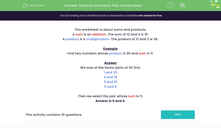# Products and Sums: Find Two Numbers

In this worksheet, students select numbers whose product and sum are both given.Key stage:  KS 2

Curriculum topic:   Number: Multiplication and Division

Curriculum subtopic:   Solve Multiplication/Division Problems

Popular topics:   Ratio worksheets

Difficulty level:#### Worksheet Overview

This worksheet is about sums and products.

A sum is an addition. The sum of 12 and 3 is 15.

A product is a multiplication. The product of 12 and 3 is 36.

Example

Find two numbers whose product is 30 and sum is 11.

We look at the factor pairs of 30 first.

1 and 30

2 and 15

3 and 10

5 and 6

Then we select the pair whose sum is 11.